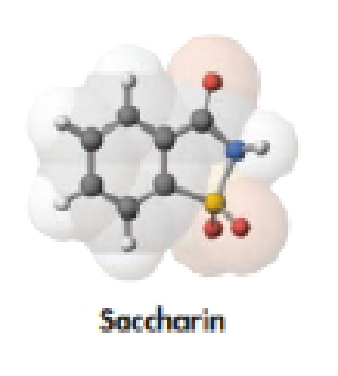# Saccharin, a molecular model of which is shown below, is more than 300 times sweeter than sugar. It was first made in 1807, when it was common practice for chemists to record the taste of any new substances they synthesized. (a) Write the molecular formula for the compound, and draw its structural formula. (S atoms are yellow.) (b) If you ingest 125 mg of saccharin, what amount (moles) of saccharin have you ingested? (c) What mass of sulfur is contained in 125 mg of saccharin?### Chemistry & Chemical Reactivity

9th Edition
John C. Kotz + 3 others
Publisher: Cengage Learning
ISBN: 9781133949640

#### Solutions

Chapter
Section### Chemistry & Chemical Reactivity

9th Edition
John C. Kotz + 3 others
Publisher: Cengage Learning
ISBN: 9781133949640
Chapter 2, Problem 125GQ
Textbook Problem
18 views

## Saccharin, a molecular model of which is shown below, is more than 300 times sweeter than sugar. It was first made in 1807, when it was common practice for chemists to record the taste of any new substances they synthesized.(a) Write the molecular formula for the compound, and draw its structural formula. (S atoms are yellow.)(b) If you ingest 125 mg of saccharin, what amount (moles) of saccharin have you ingested?(c) What mass of sulfur is contained in 125 mg of saccharin?(a)

Interpretation Introduction

Interpretation: For the given molecular model of saccharin, the molecular formula, structural formula and its structural formula are needed to be determined.

Concept introduction:

• Molecular formula of compound is the representation with symbols of each atom and their numbers present in the compound.
• Structural formula of compound is the representation of all the atoms and their respective bonding in a plane.

### Explanation of Solution

In the given model of saccharin, there are C atoms, 5 H atoms, 1 N atom, 3 O atoms and 1 S atom.

Therefore, the molecular formula of saccharin is C7H5N

(b)

Interpretation Introduction

Interpretation: The number of moles saccharin in 125g is needed to be determined.

Concept introduction:

Moles: One mole is equivalent to the mass of the substance consists same number of units equal to the atoms present in 12g of 12C.

From given mass of substance moles could be calculated by using the following formula,

Molesofsubstance GivenmassofsubstanceMolecularmass

(c)

Interpretation Introduction

Interpretation: The mass of sulfur present in give amount of saccharin should be determined.

Concept introduction:

Moles: One mole is equivalent to the mass of the substance consists same number of units equal to the atoms present in 12g of 12C.

From given mass of substance moles could be calculated by using the following formula,

Molesofsubstance GivenmassofsubstanceMolecularmass

### Still sussing out bartleby?

Check out a sample textbook solution.

See a sample solution

#### The Solution to Your Study Problems

Bartleby provides explanations to thousands of textbook problems written by our experts, many with advanced degrees!

Get Started

Find more solutions based on key concepts
Legumes are a particularly nutritious choice among protein-rich foods because they also provide a. vitamin C an...

Nutrition: Concepts and Controversies - Standalone book (MindTap Course List)

What are sister chromatids?

Human Heredity: Principles and Issues (MindTap Course List)

Give the common name and major uses of the simplest alkyne.

Chemistry for Today: General, Organic, and Biochemistry

Is it possible to have damped oscillations when a system is at resonance? Explain.

Physics for Scientists and Engineers, Technology Update (No access codes included)

Why do you suppose a male attaches permanently to the female in this species?

Oceanography: An Invitation To Marine Science, Loose-leaf Versin